Gamma-distribution

(diff) ← Older revision | Latest revision (diff) | Newer revision → (diff)

A continuous probability distribution concentrated on the positive semi-axis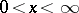with density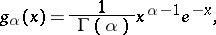whereis a parameter assuming positive values, and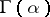is Euler's gamma-function:The corresponding distribution function for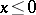is zero, and for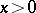it is expressed by the formula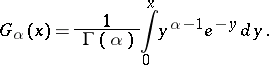The integral on the right-hand side is called the incomplete gamma-function. The density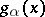is unimodal and for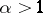it attains the maximum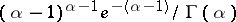at the point. If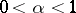the densitydecreases monotonically with increasing, and if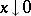,increases without limit. The characteristic function of the gamma-distribution has the form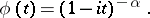The moments of the gamma-distribution are given by the formula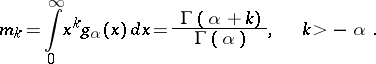In particular, the mathematical expectation and variance are equal to. The set of gamma-distributions is closed with respect to the operation of convolution: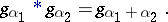Gamma-distributions play a significant, though not always an explicit, role in applications. In the particular case ofone obtains the exponential density. In queueing theory, the gamma-distribution for anwhich assumes integer values is known as the Erlang distribution. In mathematical statistics gamma-distributions frequently occur owing to the close connection with the normal distribution, since the sum of the squaresof independent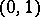normally-distributed random variables has density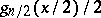and is known as the "chi-squared" distribution withdegrees of freedom. For this reason the gamma-distribution is involved in many important distributions in problems of mathematical statistics dealing with quadratic forms of normally-distributed random variables (e.g. the Student distribution, the Fisher-distribution and the Fisher-distribution). If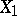andare independent and are distributed with densitiesand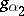, then the random variable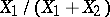has density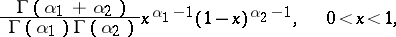which is known as the density of the beta-distribution. The densities of linear functionsof random variablesobeying the gamma-distribution constitute a special class of distributions — the so-called "type III" family of Pearson distributions. The density of the gamma-distribution is the weight function of the system of orthogonal Laguerre polynomials. The values of the gamma-distribution may be calculated from tables of the incomplete gamma-function , .